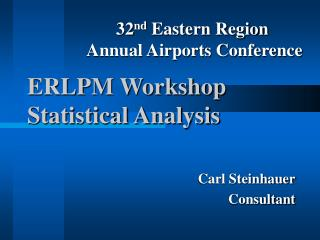DownloadDownload PresentationERLPM Workshop Statistical Analysis

# ERLPM Workshop Statistical Analysis

Télécharger la présentation## ERLPM Workshop Statistical Analysis

- - - - - - - - - - - - - - - - - - - - - - - - - - - E N D - - - - - - - - - - - - - - - - - - - - - - - - - - -
##### Presentation Transcript

1. 32nd Eastern Region Annual Airports Conference ERLPM WorkshopStatistical Analysis Carl Steinhauer Consultant

2. Limit # of samples Statistical Analysis Estimate average and % within limits Analysis % Taller than 5’-5” % between 5’-5” and 6’-5” Average Height

3. Overview P 401 Test Results Statistical Analysis; PWL Estimate Verify Production Process: Payment

4. Theory • Assumptions • Normal Distribution • Tools: Average and Standard Deviation • Percent Within Limits (PWL) Concept

5. Assumptions • Limited # of test results Statistical Analysis Quality characteristics of large amount of material • Test result variability Components: materials sampling-ERLPM testing-ERLPM • Same Process • Random sampling-Lot, Sublot • Normal Distribution

6. Specific Procedures • Sublots, Lots, Partial Lots • Calculations • Retesting • Outliers

7. 96 tests n=100

8. x x+/- 1Sn=68% x+/-2Sn+95% x+/-3Sn+99.7%

9. PWL-% of test result exceeding L L L=spec lower tolerance limit eg. Mat density 96.3 for P401

10. PWL Calculation ProceduresERLPM-page 47 Section 110-AC 1505370-10C Method for Computing PWL and Examples Section 110-02 Spec. P401 Table 5: L and U Spec. Limits (page 24)

11. Table 5: Marshall Acceptance Limits

12. Given x1=2 x2=4 x3=6 x4=8 X = 2+4+6+8 = 5 4

13. X=5 d1=2-5=-3 d1²=9 d2=4-5=-1 d2²=1 d3=6-5=1 d3²=1 d4=8-5=3 d4²=9 Sn= d1²+d2²+d3²=d4² n-1 Sn = 9+1+1+9 = 20 4-1 3 Sn =2.58 (calculator n-1)

14. Roundout RulesERLPM-page 47 Example-last digit to be kept-nearest 10th 4.61 4.62 4.64 4.6500 4.66 4.67 4.68 4.69 Even Digit-same Odd Digit-increase by 1 This case becomes 4.6 If it was 4.7500 it would become 4.8 becomes 4.7

15. MAT Density –One side density acceptance (Manual Appendix E, page 4) Sublot 1. = 96.0 2. = 97.0 3. = 99.0 4. = 100.0 x=98.0 Sn=1.8 QL=x-L Sn QL=98.0-96.3 = .9444 1.8 Section 110-Table l, N=4 PL=82 Section 110-02f Spec Tables 5 page 50 ERLPM

16. Mat Density -Two sided acceptance for density x=98.0 Sn=1.8 Qu=L-x Sn QL=101.3-98.0 = 1.833 1.8 Section 110-Table l, N=4 PL= 100

17. PWL calculation for two sided specification PWL= PL + PU-100 PWL= 82 + 100 – 100 = 82

18. MAT Density PL = 82% Target Density 98.0 Achieved Sn=1.8 versus 1.3 98.0 18% 96.3 Acceptable QC Value

19. MAT Density PL = 82% Target Density 98.0 Achieved Sn=1.8 versus 1.3 98.0 18% 96.3 101.3% Acceptable QC Value

20. Effect of Quality Control Sn = 1.3 Spec. pg 24 Sn = 1.8 82 PWL 90 PWL 96.3% 98% x

21. Air VoidsApp. D, page 3 Sublot 1= 2.1 2= 3.2 3=2.5 4=6.0 X= 3.4 Sn= 1.76 Spec. page 5 - 0.65

22. QL = x-L = 3.4-2.0 = .7955 Sn 1.76 PL(table 1) = 77% n=4 QU= U-x = 5-3.4 = .909 Sn 1.76 PU(table 1) = 81% n=4 PWL= PL + PU-100 PWL= 77+81-100 = 58

23. Air Voids 58% 23% 19% 2 5 3.4 PWL= PL + PU-100 PWL= 77 + 81-100 =58

24. Payment – One side for density TABLE 6. PRICE ADJUSTMENT SCHEDULE 1

25. Payment – two sided for density TABLE 6. PRICE ADJUSTMENT SCHEDULE 1

26. Payment Spec-par 401-8.1a-page 29 MAT Density PWL=82 Air Voids PWL= 58 Lot Pay Factor Air Voids- 1.4 x 58-12= 69.2% Mat Density- 0.5 x 82+55= 96% Use lower of 2 values- 69.2% Lower value

27. Joint DensityAppendix E, page 5 93.3 95.0 97.0 96.0 X= 95.3 Sn= 1.58 QL= (95.3-93.3) = 1.2658 1.58 PL= 93 Spec. page 21 par. 401-5.2(b)(3) if < 71% there is a 5% penalty Table 5

28. Partial Lotsspec page 20 Section P-401-5.1c

29. Sample Problem Flow-Appendix D, page 5 Partial lot situation-6 sublots 8.0, 8.2, 8.5, 8.2, 8.9, 9.1 X= 8.5 Sn= 0.44 QL= x-L = 8.5-8.0 = 1.1364; PL= 88 Sn 0.44 QU= U-X = 16-8.5 = 18.75; PL= 100 Sn 0.44 PWL= 88 + 100-100= 88<90 Corrective Action! 401-5.2(b)(2)

30. Outliers Spec -pg 23 401-5.2d -pg 25 401-5.3c MAT Density and Air Voids

31. Test for Outliers MAT Density 94.0 96.0 97.0 98.0 x= 96.2 Sn= 1.71 QL= 96.2-96.3 = -.0585 1.71 PL= <50% ASTM E 178, par. 4 T1= (x-x1)/Sn T1= 96.2-94 = 1.286 1.71

32. Table 1-ASTM E 178 • N=4 • Upper 5% significance level 1.463 • Since 1.286<1.463 the 94.0 test value is not considered an outlier and is retained!

33. Sample Problem-Outliers Air Voids 2.0, 4.8, 4.9, 5.0 X=4.2 Sn=1.45 QL= 4.2-2.0 = 1.5172; PL= 100 1.45 QU= 5-4.2 = 0.5517; PU= 69 1.45 PWL= (100+69)-100=69 ASTM E 178 par. 4 Tn= (x-x1)/Sn = 4.2-2.0 = 1.517 1.45 Table 1, ASTM E 178, N=4, 5% significance T= 1.463<1.517 therefore 2.0 is the outlier and it is discarded

34. Resampling401-5.3 page 25 MAT Density ONLY (Appendix E-pg 4) Prior MAT Density- 96, 97, 99, 100 PWL 82 4 new cores 96, 96, 97, 98 AVG-all 8, 97.4 Sn= 1.51 QL= x-L = 97.4-96.3 = .7337 Sn 1.51 Table 1, N= 8 PWL= 77

35. Questions?

36. Thanks!